# Matplotlib Series 7: Area chart

This blog specifies how to create simple area charts, multiple area charts, stacked area charts and 100% stacked area charts with matplotlib in Python, and their use cases.

This blog is part of Matplotlib Series:

## Area chart

An area chart or area graph displays graphically quantitative data. It is based on the line chart. The area between axis and line are commonly emphasized with colors, textures and hatchings.

### When to use it ?

• Show or compare a quantitative progression over time.

### Example 1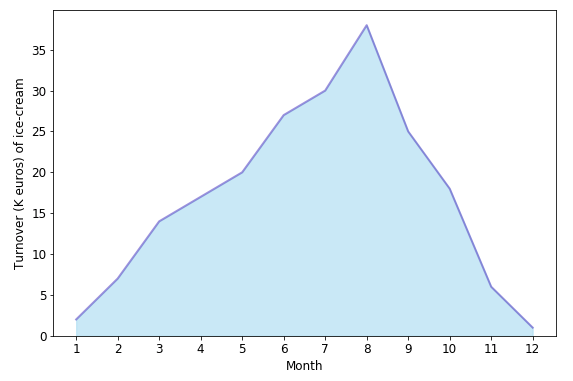Suppose that the plot above describes the turnover(k euros) of ice-cream’s sales during one year. According to the plot, we can clearly find that the sales reach a peak in summer, then fall from autumn to winter, which is logical.

### Example 2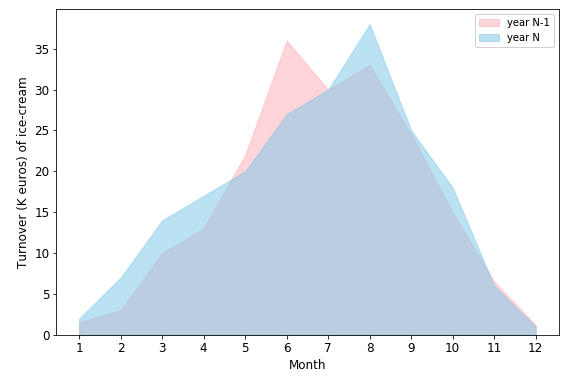As an extension of the first plot, the second one compares two-year turnover together. The peak for both year is in the summer, for year N, the peak is in August; however, the peak is reached in June in the year N-1, which is caused by the heatwave in June.

## Stacked area chart

Stacked Area Graphs work in the same way as simple Area Graphs do, except for the use of multiple data series that start each point from the point left by the previous data series.

### When to use it ?

• Represent cumulated totals using numbers or percentages over time.
• Visualize part-to-whole relationships, helping show how each category contributes to the cumulative total.

### Example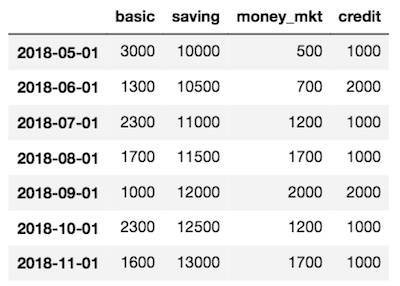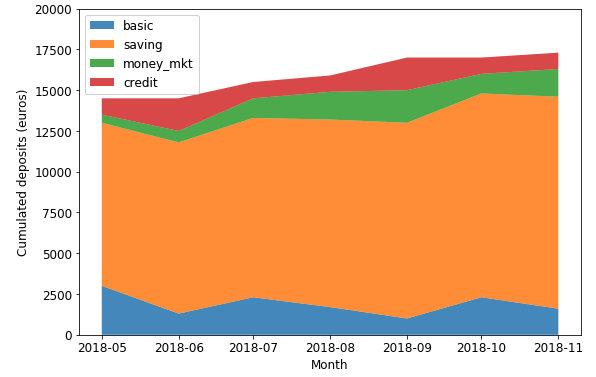This stacked area chart displays the amounts’ changes in each account, their contribution to total amount (in term of value) as well.

## 100% Stacked Area Chart

Data plotted as areas and stacked so that the cumulative area always represents 100%.

### When to use it ?

• This is best used to show distribution of categories as parts of a whole, where the cumulative total is unimportant.

### Example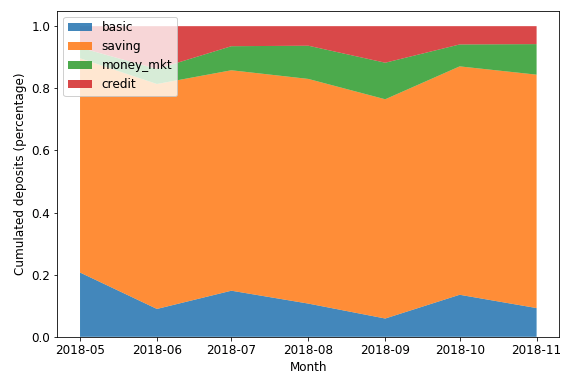This stacked area chart displays the amounts’ changes in each account, their contribution to total amount (in term of percentage) as well.